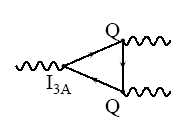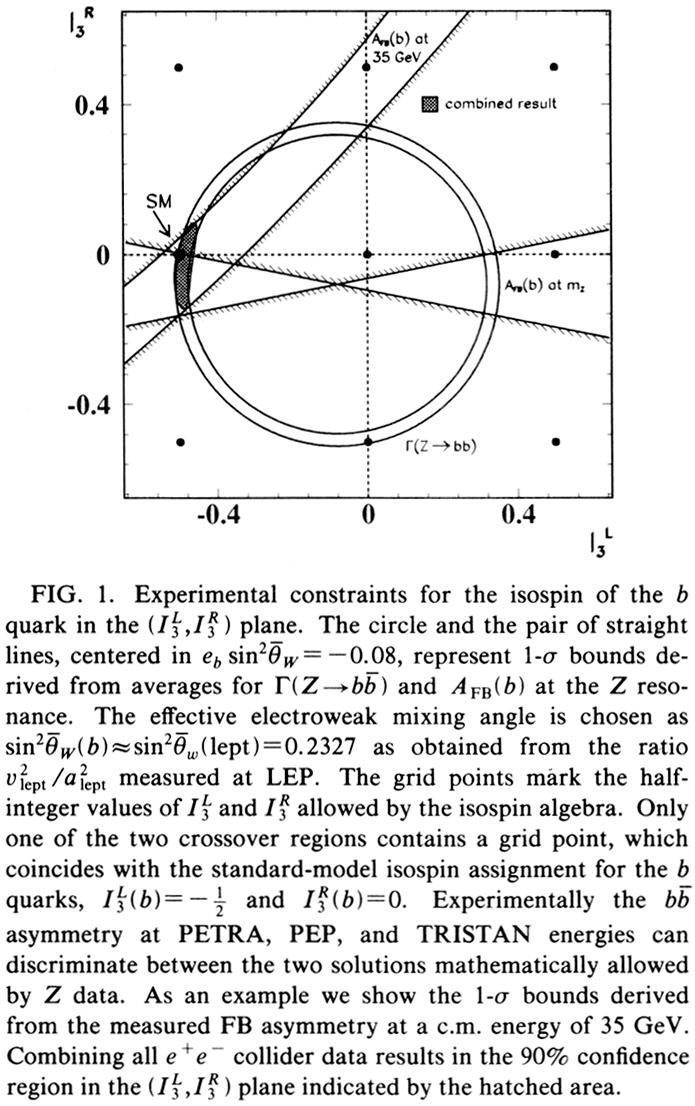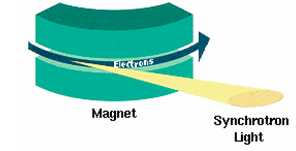## Top quark: a short history – part II November 17, 2007

Posted by dorigo in physics, science.

Compelling theoretical evidence for the top quark

The spectacular experimental results mentioned in the first section of this multi-part post were the preludes to the searches of the top quark which were forthcoming in the eighties and nineties. The top quark had to be there: the elegance of the Standard Model and the presence of CP violation in weak interactions strongly hinted at the existence of a sixth quark, and so did also the existence of the tau lepton and b quark. But even more compelling were three independent theoretical arguments.1. The Standard Model is not a renormalizable gauge theory in the absence of the top quark. Renormalizability is a crucial feature, enabling the SM to be theoretically consistent and be usable as a tool to compute the rate of subnuclear processes between quarks, leptons, and gauge bosons. Diagrams containing so-called “triangle anomalies” like the one shown on the right, where an axial-vector current couples to two vector currents, cancel their contribution to any process, and thus avoid breaking the renormalizability of the SM,  only if the sum of electric charges of all fermions circulating in the triangular loop is zero:$\Sigma Q = -1 + 3 \times [2/3 + (-1/3)]=0$, where -1 is the electric charge of leptons, and 2/3, 1/3 are the charges of up- and down-type quarks, while the additional factor of three accounts for the three colours of each quark. It is evident that each complete generation of left-handed fermions has a zero sum of electric charges, while an incomplete third generation -one with a tau, a tau neutrino, three b-quarks, and no up-type partners of the b-quarks – would contribute a non-zero total charge: triangle anomalies would thus make the SM a useless, non-renormalizable, illogic construct.

By the way, since I am discussing anomaly cancellation, I want to submit the most knowledgeable of my readers some thoughts connected to the issue, in the hope of getting some meaningful input. I am intrigued by the following fascinating fact: if you were some deity and you were constructing the standard model from scratch, one could argue you would care for renormalizability – it would allow you to compute stuff in a simple mathematical framework. Then, having introduced doublets of quarks and leptons -you need both to construct molecular structures-, you would be facing the choice on the number of colors of quarks (which you need in order to provide stability to nuclear matter, as well as to satisfy the antisymmetric form of the total wavefunction of baryons) and the simultaneous choice of their electric charge.  These are bound to satisfy the rule$\Sigma Q = -1 + N_c \times (Q_u + Q_d)=0$ in order to cancel axial-vector-vector anomalies, as well as the requirement that$Q_u-Q_d=1$ (so that W bosons allow charged current interactions between quarks as well as leptons). You would be hard pressed to choose$N_c$ any different from three if you wanted to build integer-charge mesons and baryons from$q \bar q$ and$qqq$ states! That follows from the fact that the two relations above imply$N_c=\frac{1}{1+2Q_d}$. Now, with$N_c=1$ you have no strong force with anti-screening, while if you take$N_c=2$ you cannot build 3-quark states any more – non-integer charge and non-zero color! Of course, you could still make 2-quark and 4-quark states, but I am not sure one could then achieve stable matter any more. If you know some literature on the topic of building a consistent, alternative version of the SM with different quark charges, I would be glad to get a reference in the comments section below. Forget the large Nc limit though…

2. The smallness of flavor-changing neutral current decays of hadrons, of which the$K^0 \to \mu \mu$ decay mentioned in part I is an example, demands the existence of a partner for the bottom quark, in much the same way as the charm quark is needed by the GIM mechanism discussed above. For b quarks, the relevant decay is$B^0 \to \mu \mu$, which has escaped detection this far due to its extreme rarity.3. The UA1 experiment, soon followed by the ARGUS and CLEO experiments,  measured in the late eighties the phenomenon called flavor oscillation in the system of the neutral B mesons. This topic would require a post by itself, but in short, neutral B mesons, particles made by a$b \bar d$, can transform into their own antiparticles$\bar b d$ through box diagrams involving the exchange of two weak bosons, like the one shown in the picture (which describes the mixing of$B_s$ mesons rather than$B_d$ ones, but the very same principle is at work). The rate of the oscillation, measured by the experiments, implied that the top quark existed, providing a contribution to the diagrams. These studies also implied that the top quark mass had to be larger than about 50 GeV already in 1987.

4. The phenomenology of certain electroweak processes critically depends on an attribute of the bottom quark called weak isospin. This is a number equal to$I^L_3 = \pm 1/2$ for left-handed fermions belonging to a doublet, such as$(u d)_L$ or$(c s)_L$, and equal to zero for singlets, such as the right-handed fermions. If left-handed b quarks were lonely isosinglets, the rate of several processes mediated by weak neutral currents would be quite different. In the eighties and early nineties b-quarks were studied at electron-positron machines running at energies below the Z mass (PEP, PETRA, TRISTAN), and then Z bosons were studied at the Large Electron Positron collider at CERN. From asymmetries in b quark production at lower energy and from the rate and angular characteristics of Z decays to b-quark pairs measured at LEP, the weak isospin of left-handed bottom quarks was determined to be exactly$I^L_3=-1/2$: the bottom quark was thus recognized as the lower component of a weak isodoublet.

In the picture below, which retains the original caption of the paper by Schaile and Zerwas (PRD 45 (1992), p.3262) you can see a lattice of possible values of the right-handed and left-handed weak isospin of the b-quark, and regions of the plane allowed by measurements of the rate of$Z \to b \bar b$ decays (the circle), the forward-backward asymmetry in$b \bar b$ production by PETRA (the small swath cutting diagonally the plane) and same measurements at the Z pole (the hourglass-like region cutting the plane horizontally). The three allowed regions meet at$I^R_3=0, I^L_3=-1/2$.The top quark could not escape such a mountain of evidence any more! Few physicists doubted that the top quark was really there. And yet, the massive particle would not show up until the next decade…

Searches for the top quark: the tools

As previous experience had shown, particle physicists had two main avenues to search for a new massive quark: electron-positron annihilations or hadron-hadron collisions. A tie had resulted from the competition of the two approaches in the charm discovery of 1974, when the former had been successfully used by Burton Richter’s team at the SLAC laboratory, while the latter had been exploited by Samuel Ting and his collaborators at Brookhaven. In the case of the bottom quark, however, hadron collisions had gotten there first. So the question at the end of the seventies was: which of the two approaches is going to win the race to the top quark ? An engineering concept called scalability was going to drive the decision on where to place one’s bet.

Electron-positron annihilations provide by far a cleaner, easier-to-interpret signature: all the energy of the two projectiles is available in the final state, and it just becomes a matter of reaching the threshold where energy is sufficient to create the new particle and its antimatter companion,$E > 2 m_q$ (E is total collision energy,$m_q$ the quark mass): right there and then, the rate of collisions yielding hadrons shows an increase, and the final state may exhibit striking new signatures.The main problem with electron-positron colliders lies, indeed, in scalability, that is the issue related to increasing the size and power of accelerators in order to reach higher energies. If you bend the trajectory of an electron moving at relativistic speed, it will radiate some of its energy by the process called synchrotron radiation (see figure). The power P needed to maintain the electron’s energy E in a circular machine increases with the steep law$P=E^4$, and it rapidly becomes forbidding. One is thus left with two options: increase the radius of curvature of the accelerator, or build a longer linear collider tightly packed with accelerating cavities. Both these options were used respectively at CERN and SLAC in the late eighties, reaching the threshold for Z production (and ten years later doubling it with LEP II). But the top quark was far too heavy for these machines, unfortunately.

Hadron collisions are way murkier than clean electron-positron annihilations: a hard collision between a pair of quarks is invariably accompanied by a messy final state caused by the debris of the remnants – the other constituents of the projectiles. Their advantage is that hadron colliders are less affected by scalability issues such as those discussed above. In particular, synchrotron radiation is irrelevant for protons, since it is reduced by a factor of$(m_p/m_e)^4$, because the radiated energy is inversely proportional to the fourth power of the particle mass.

However, one has to first of all realize that the strategy of creating an intense, energetic beam of protons and smashing it against a thin target is an inefficient way to produce high-energy collisions: a few basic formulas of relativistic kinematics can show that the center-of-mass energy of a collision between a moving proton of energy E and another in a fixed target is$E^{CM} _{f.t.} =\sqrt { 2 E m_p }$, while for a head-on collision between two protons of energy E’ one gets$E^ {CM} _ {head-on} =2E'$. The square root in the first expression is a true killer: since$m_p \sim 1 GeV$, if you want a center-of-mass energy of 10 GeV (such that you can in principle produce pairs of 5-GeV particles) you need about E=50 GeV energy for the beam hitting the fixed target, which may seem not too much higher than the equivalent E’=5 GeV of each intersecting beams in a collider; but if you want 100 GeV in the center of mass, you need no less than 5000 GeV hitting the fixed target as opposed to only 50 for the colliding beams!

Having realized that to discover massive states with hadron collisions one really needs to produce head-on collisions among hadrons traveling at high speed in opposite directions, there remains a choice to make: protons against protons, or protons against antiprotons ? Antiprotons are way, way, way harder to produce than protons! You need to accelerate a proton to high energy, direct it to a fixed target, and sift through the stream of particles produced by the collision downstream, selecting with suitable magnets antiprotons as particles having negative charge and a mass of about 0.938 GeV. The typical efficiency to produce and collect antiprotons this way is one in a hundred thousand to one in a million: you need a million proton collisions to get a few antiprotons!

Antiprotons are indeed a rare merchandise. However, if you manage to put together a beam of antiprotons, you can inject it in the same synchrotron that circulates the beam of protons, because the opposite charge of the two beams allows them to travel in the same vacuum structure and bend the right way under the action of the same magnetic dipoles!

The economy of having to build only one synchrotron rather than two parallel ones with separate magnets proved more valuable than the difficulty in producing antiproton beams. At CERN a 546 GeV proton-antiproton collider (later upgraded to 630 GeV) was built and allowed the UA1 and UA2 experiments to successfully discover the W and Z bosons, as already mentioned. Meanwhile, at Fermilab the 400 GeV proton synchrotron with which the b-quark had been discovered was converted into a 1800 GeV proton-antiproton collider.

The race was on. The main goal of the$Sp \bar p S$ was to discover weak gauge bosons, while the Tevatron, which was trailing by several years, aimed directly at the top quark discovery. It turned out that the latter was lucky: a top quark lighter than 60 GeV or so would have certainly become sweet icing on the cake of the CERN experiments’ achievements, but the top was way out of the UA1 and UA2 experiments reach.

[to  be continued…]